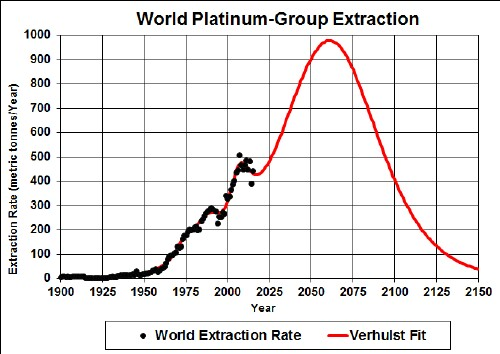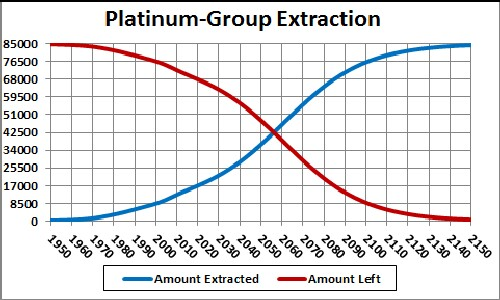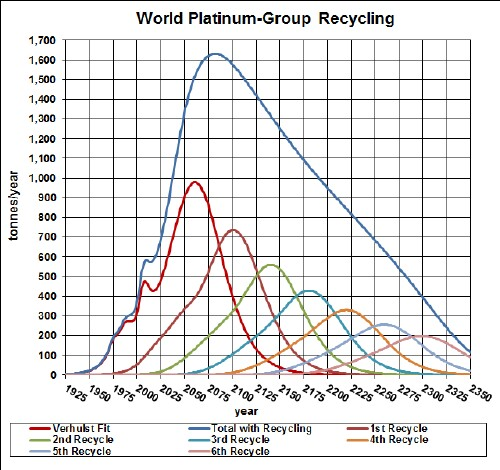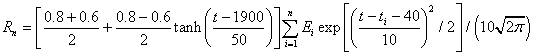# Platinum-Group Depletion Including Recycling

Platinum is used extensively in electronics manufacturing and as a catylist.

The graph below shows the platinum-group extraction data for the world and Verhulst function fits to the data for two different amounts of eventual extraction in order to extrapolate into the future.Platinum-group extraction rate for the world and several Verhulst functions fit to the data.

The red curve is obtained by restricting the total extraction (85x103 tonnes) to the amount already extracted (~1.4x103 tonnes) plus the estimated reserves base of about 66x103 tonnes.

Taking an average extraction curve of the two fits, the crossover point at year ~2058 when the amount extracted is equal to the amount left to be extracted is shown here:## Recycling

Undoubtedly there is much recycling of platinum-group, especially since many people and nations hold platinum-group as a "currency". By the term "recycle" I refer to platinum-group that is resold for storage as well as to be used to make items.

Assume that:

• The platinum-group-extraction curve is the average of the two curves given above.
• Recycling of platinum-group follows a hyperbolic tangent curve from 60% to 80% recycling with a break point of year 1900 and width 50 years.
• The recycling is delayed by a Gaussian curve peaking at a delay of 40 years and a width of 10 years.

The effective platinum-group available for purchase after the first ten recycling cycles is shown in the following graph, along with the effective platinum-group available for each cycle for the average of the two extraction cases described above:The equation for a recycling cycle is,

where Ei is the amount available from the previous cycle. Here is an example of the Excel coding:

{=((\$J\$2+\$I\$2)/2+((\$J\$2-\$I\$2)/2)*TANH((A27-\$K\$2)/\$L\$2))*SUM(\$I\$27:I27*(EXP(-1*((A27-\$A\$27:A27-\$N\$2)/\$O\$2)^2/2))/\$O\$2/SQRT(2*PI()))} (The curly bracket surrounding the term makes it into an array; it must be entered by holding down the SHIFT & CTRL keys while pressing the ENTER key.)

Of course, the recycling could be extended to more cycles, skewing the curve further into the future. However, the peak and fall off after it will not change because further cycles are essentially zero in that time region.

Thus, under the assumptions given above, the effective amount of platinum-group available for making items peaks before 2125 and falls off rapidly after that. Humans will have taken concentrated platinum-group deposits and scattered them across the surface of the earth.

The Excel spreadsheet is set up to make it easy to calculate with different recycling assumptions.

Minerals Depletion